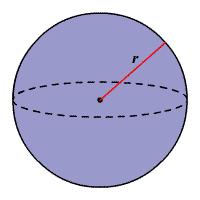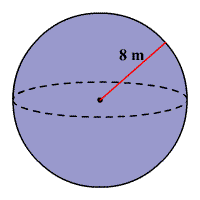# Volume of a Sphere

A sphere is a set of points in space that are a given distance $r$ from the center.The volume of a $3$ -dimensional solid is the amount of space it occupies.  Volume is measured in cubic units( ${\text{in}}^{3},{\text{ft}}^{3},{\text{cm}}^{3},{\text{m}}^{3}$ , et cetera).  Be sure that all of the measurements are in the same unit before computing the volume.

The volume $V$ of a sphere is four-thirds times pi times the radius cubed.

$V=\frac{4}{3}\pi {r}^{3}$

The volume of a hemisphere is one-half the volume of the related sphere.

Note : The volume of a sphere is $2/3$ of the volume of a cylinder with same radius, and height equal to the diameter.

Example:

Find the volume of the sphere. Round to the nearest cubic meter.Solution

The formula for the volume of a sphere is

$V=\frac{4}{3}\pi {r}^{3}$

From the figure, the radius of the sphere is $8$ m.

Substitute $8$ for $r$ in the formula.

$V=\frac{4}{3}\pi {\left(8\right)}^{3}$

Simplify.

$V=\frac{4}{3}\pi \left(512\right)$

$\approx 2145$

Therefore, the volume of the sphere is about $2145\text{\hspace{0.17em}}{\text{m}}^{3}$ .